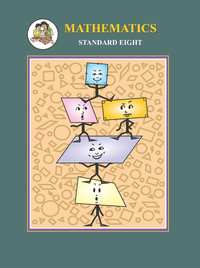# Balbharati solutions for Mathematics 8th Standard Maharashtra State Board chapter 5 - Expansion formulae [Latest edition]

#### Chapters## Chapter 5: Expansion formulae

Practice Set 5.1Practice Set 5.2Practice Set 5.3Practice set 5.3Practice Set 5.4
Practice Set 5.1 [Page 24]

### Balbharati solutions for Mathematics 8th Standard Maharashtra State Board Chapter 5 Expansion formulae Practice Set 5.1 [Page 24]

Practice Set 5.1 | Q 1 | Page 24

Expand.

(a+2)(a−1)

Practice Set 5.1 | Q 2 | Page 24

Expand.

(m−4)(m+6)

Practice Set 5.1 | Q 3 | Page 24

Expand.

(p+8) (p−3)

Practice Set 5.1 | Q 4 | Page 24

Expand.

(13+x) (13−x)

Practice Set 5.1 | Q 5 | Page 24

Expand.

(3x+4y) (3x+5y)

Practice Set 5.1 | Q 6 | Page 24

Expand.

(9x−5t) (9x+3t)

Practice Set 5.1 | Q 7 | Page 24

Expand.

(m+2/3)(m-7/3)

Practice Set 5.1 | Q 8 | Page 24

Expand.

(x+1/x)(x-1/x)

Practice Set 5.1 | Q 9 | Page 24

Expand.

(1/y+4)(1/y-9)

Practice Set 5.2 [Page 25]

### Balbharati solutions for Mathematics 8th Standard Maharashtra State Board Chapter 5 Expansion formulae Practice Set 5.2 [Page 25]

Practice Set 5.2 | Q 1 | Page 25

Expand

(k + 4)3

Practice Set 5.2 | Q 2 | Page 25

Expand

(7x + 8y)3

Practice Set 5.2 | Q 3 | Page 25

Expand

(7 + m)3

Practice Set 5.2 | Q 4 | Page 25

Expand

(52)3

Practice Set 5.2 | Q 5 | Page 25

Expand

(101)3

Practice Set 5.2 | Q 6 | Page 25

Expand

(x + 1/x)^3

Practice Set 5.2 | Q 7 | Page 25

Expand

(2m + 1/5)^3

Practice Set 5.2 | Q 8 | Page 25

Expand

((5x)/y + y/(5x))^3

Practice Set 5.3, Practice set 5.3 [Page 27]

### Balbharati solutions for Mathematics 8th Standard Maharashtra State Board Chapter 5 Expansion formulae Practice Set 5.3, Practice set 5.3 [Page 27]

Practice Set 5.3 | Q 1.1 | Page 27

Expand

(2m − 5)3

Practice Set 5.3 | Q 1.2 | Page 27

Expand

(4 − p)

Practice Set 5.3 | Q 1.3 | Page 27

Expand

(7x − 9y)3

Practice Set 5.3 | Q 1.4 | Page 27

Expand

(58)3

Practice Set 5.3 | Q 1.5 | Page 27

Expand

(198)3

Practice Set 5.3 | Q 1.6 | Page 27

Expand

(2p - 1/(2p))^3

Practice Set 5.3 | Q 1.7 | Page 27

Expand

(1 - 1/a)^3

Practice Set 5.3 | Q 1.8 | Page 27

Expand

(x/3 - 3/x)^3

Practice Set 5.3 | Q 2.1 | Page 27

Simplify

(2a + b)− (2a − b)3

Practice Set 5.3 | Q 2.2 | Page 27

Simplify

(3r − 2k)3 + (3r + 2k)3

Practice Set 5.3 | Q 2.3 | Page 27

Simplify

(4a − 3)− (4a +3)3

Practice set 5.3 | Q 2.4 | Page 27

Simplify

(5x − 7y)+ (5x + 7y)3

Practice Set 5.4 [Page 28]

### Balbharati solutions for Mathematics 8th Standard Maharashtra State Board Chapter 5 Expansion formulae Practice Set 5.4 [Page 28]

Practice Set 5.4 | Q 1.1 | Page 28

Expand.

(2p + q +5)2

Practice Set 5.4 | Q 1.2 | Page 28

Expand.

(m + 2n +3r)2

Practice Set 5.4 | Q 1.3 | Page 28

Expand.

(3x +4y − 5p)2

Practice Set 5.4 | Q 1.4 | Page 28

Expand.

(7m − 3n − 4k)2

Practice Set 5.4 | Q 2.1 | Page 28

Simplify.

(x − 2y +3)2 + (x + 2y − 3)2

Practice Set 5.4 | Q 2.3 | Page 28

Simplify.

(3k − 4r − 2m)− (3k + 4r − 2m)2

Practice Set 5.4 | Q 2.3 | Page 28

Simplify.

(7a − 6b + 5c)+ (7a + 6b − 5c)2

## Chapter 5: Expansion formulae

Practice Set 5.1Practice Set 5.2Practice Set 5.3Practice set 5.3Practice Set 5.4## Balbharati solutions for Mathematics 8th Standard Maharashtra State Board chapter 5 - Expansion formulae

Balbharati solutions for Mathematics 8th Standard Maharashtra State Board chapter 5 (Expansion formulae) include all questions with solution and detail explanation. This will clear students doubts about any question and improve application skills while preparing for board exams. The detailed, step-by-step solutions will help you understand the concepts better and clear your confusions, if any. Shaalaa.com has the Maharashtra State Board Mathematics 8th Standard Maharashtra State Board solutions in a manner that help students grasp basic concepts better and faster.

Further, we at Shaalaa.com provide such solutions so that students can prepare for written exams. Balbharati textbook solutions can be a core help for self-study and acts as a perfect self-help guidance for students.

Concepts covered in Mathematics 8th Standard Maharashtra State Board chapter 5 Expansion formulae are Expansion of (x + a)(x + b), Expansion of (a + b)3, Expansion of (a - b)3, Expansion of (a + b + c)2.

Using Balbharati 8th Standard solutions Expansion formulae exercise by students are an easy way to prepare for the exams, as they involve solutions arranged chapter-wise also page wise. The questions involved in Balbharati Solutions are important questions that can be asked in the final exam. Maximum students of Maharashtra State Board 8th Standard prefer Balbharati Textbook Solutions to score more in exam.

Get the free view of chapter 5 Expansion formulae 8th Standard extra questions for Mathematics 8th Standard Maharashtra State Board and can use Shaalaa.com to keep it handy for your exam preparation